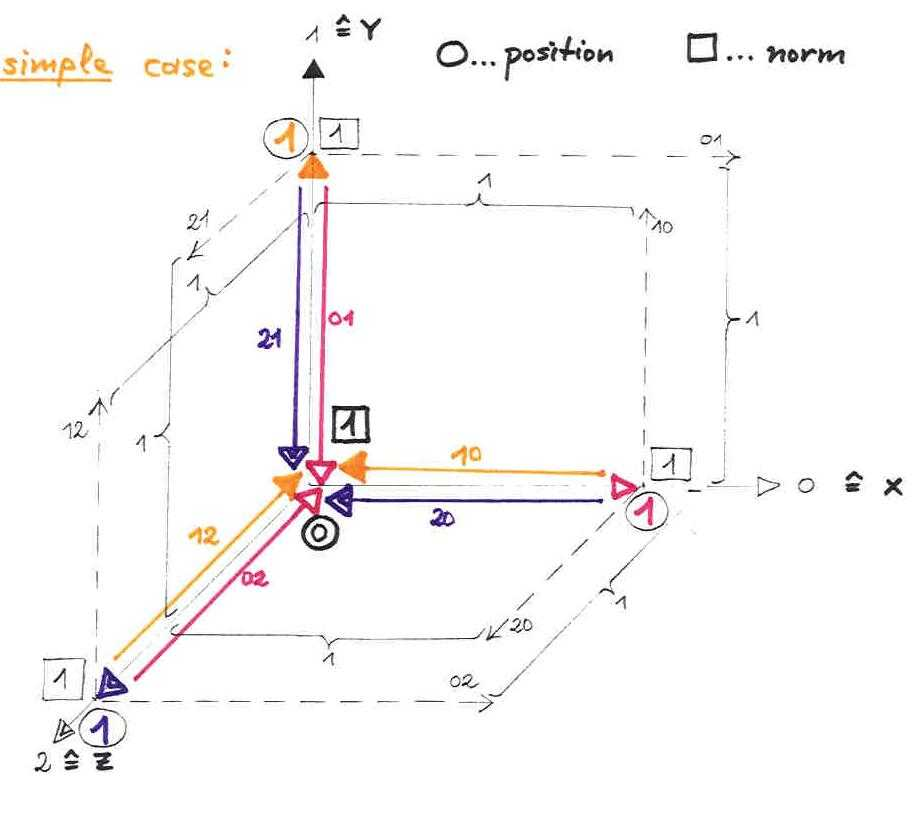# of Karim Belabas, Aïssa Derhem, and Daniel C. Mayer

 * On these pages, we present most recent results of our joint research, directly from the lab. * Basic bibliography: K. Belabas, A fast algorithm to compute cubic fields, Math. Comp. 66 (1997), 1213-1237 A. Derhem, Capitulation dans les extensions quadratiques de corps de nombres cubiques cycliques, Thèse de doctorat, Université Laval, Quebec, 1988 D. C. Mayer, Multiplicities of dihedral discriminants, Math. Comp. 58 (1992), 831-847 and S55-S58 * E-mail addresses: Karim.Belabas@math.u-psud.fr aderhem@yahoo.fr danielmayer@algebra.at *

 Dirichlet Type Lattices in Cyclic Cubic Fields (2002/04/26) Dan (02/04/26): Using VORONOI's algorithm for signature (3,0), published in his doctoral dissertation, we can determine the geometry of lattice minima in the discrete geometric image of O_L, the maximal order of any cyclic cubic field L, racing along the VORONOI HighWays in various directions. Dan (02/04/26): These lattices have the nice property that uXY = uZY = uY^-1, uYZ = uXZ = uZ^-1, uZX = uYX = uX^-1. This structure occurs with period length 1 for the conductors f = 7, 9, 13, 19, 37, 79, 97, 139, 163, 313, ..., with period length 3 for f = 43, 67, 151, 397, 541, ..., with period length 4 for f = 181, 211, 271, 421, ..., and with period length 6 for f = 127, 337, ... Chain in X-direction: no pre period v = 0, chain length pl_X = 1, N(n_X^1(1)) = 1 X-Branch in Y-direction: branch length bl_XY = 1, association on X-chain at position ap = 0, N(n_Y^1(1)) = N(n_X^0(1)) X-Branch in Z-direction: branch length bl_XZ = 1, association on X-chain at position ap = 0, N(n_Z^1(1)) = N(n_X^0(1)) The symmetry of lattices in cyclic cubic fields implies that the chains in Y-direction and in Z-direction have the same structure as the chain in X-direction.All DIRICHLET systems of units are fundamental: XY-system: index ( U : < uX,uY > ) = 1 YZ-system: index ( U : < uY,uZ > ) = 1 ZX-system: index ( U : < uZ,uX > ) = 1 which shows the symmetry again. Fundamental pairs of units are, of course, also given by: (uX,uXY),(uX,uXZ),(uY,uYZ),(uY,uYX),(uZ,uZX),(uZ,uZY).